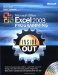# Assigning Values to Variables

 < Day Day Up >

A variable is useless if you aren't able to assign a value to it. Assigning a value to a variable is done using an equal sign (=), which is also known as the assignment operator. The name of the variable is given first, followed by the assignment operator, and then an expression of the value that you wish to assign to the variable. The value expression could be a literal value, or it could be something more complicated, such as a mathematical equation.

`MyNumber = 23YourNumber = 16 + 85MyString = "This is a literal string."TwoStrings = "This is the first part." + "This is the second part."MyDate = #05/07/1992#`

You can also assign the value from one variable to another variable, or you can reference the same variable you are assigning a value to. For example, if you are keeping a running total of hours worked, you can update the total hours worked by adding the number of hours worked during the current day.

`TotalLaps = LapCounterTotalHoursWorked = TotalHoursWorked + HoursWorkedToday`

 < Day Day Up >Microsoft Office Excel 2003 Programming Inside Out (Inside Out (Microsoft))
ISBN: 0735619859
EAN: 2147483647
Year: 2006
Pages: 161

Similar book on Amazon